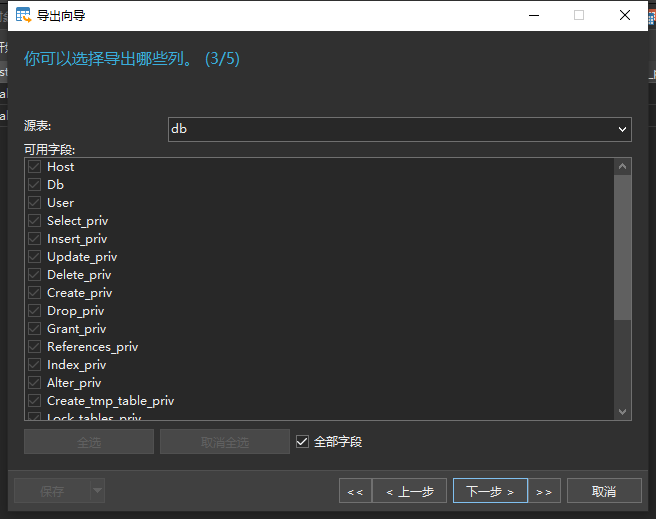# 将Mysql数据导出到Excel下载时如何下载特定字段？

If I want to change field name @ the time of excel download to submit user, how to do that? how to download particular column in the selected table?

`````` <?php
/*******EDIT LINES 3-8*******/
\$DB_Server = "localhost"; //MySQL Server
\$DB_DBName = "databasename";         //MySQL Database Name
\$DB_TBLName = "tablename"; //MySQL Table Name
\$filename = "excelfilename";         //File Name
/*******YOU DO NOT NEED TO EDIT ANYTHING BELOW THIS LINE*******/
//create MySQL connection
\$sql = "Select * from \$DB_TBLName";
\$Connect = @mysql_connect(\$DB_Server, \$DB_Username, \$DB_Password) or die("Couldn't connect to MySQL:<br>" . mysql_error() . "<br>" . mysql_errno());
//select database
\$Db = @mysql_select_db(\$DB_DBName, \$Connect) or die("Couldn't select database:<br>" . mysql_error(). "<br>" . mysql_errno());
//execute query
\$result = @mysql_query(\$sql,\$Connect) or die("Couldn't execute query:<br>" . mysql_error(). "<br>" . mysql_errno());
\$file_ending = "xls";
/*******Start of Formatting for Excel*******/
//define separator (defines columns in excel & tabs in word)
\$sep = "\t"; //tabbed character
//start of printing column names as names of MySQL fields
for (\$i = 0; \$i < mysql_num_fields(\$result); \$i++) {
echo mysql_field_name(\$result,\$i) . "\t";
}
print("
");
//end of printing column names
//start while loop to get data
while(\$row = mysql_fetch_row(\$result))
{
\$schema_insert = "";
for(\$j=0; \$j<mysql_num_fields(\$result);\$j++)
{
if(!isset(\$row[\$j]))
\$schema_insert .= "NULL".\$sep;
elseif (\$row[\$j] != "")
\$schema_insert .= "\$row[\$j]".\$sep;
else
\$schema_insert .= "".\$sep;
}
\$schema_insert = str_replace(\$sep."\$", "", \$schema_insert);
\$schema_insert = preg_replace("/
|
|
|/", " ", \$schema_insert);
\$schema_insert .= "\t";
print(trim(\$schema_insert));
print "
";
}
?>
``````

I am using Above mentioned Code to export Mysql data to Excel Download. for ex, in my table i have the fields of id, name, status, create_date.
but @ the time of export mysql data to excel download i wants to takes the filed of name & status only. help me to solve this prob. thanks for

• 点赞
• 写回答
• 关注问题
• 收藏
• 复制链接分享
• 邀请回答

#### 2条回答

• You might just be looking for a query which selects only the columns you want, something like this:

``````\$sql = "Select name as organization, status from \$DB_TBLName";
``````

instead of the following, which selects all columns:

``````\$sql = "Select * from \$DB_TBLName";
``````

Note that I used an alias to change the name of the `name` column to `organization`.

点赞 评论 复制链接分享
•导出是可以选择，要导出的字段或全部字段

点赞 评论 复制链接分享